# Time Calculator: Timesheet

## Hours Calculator Timesheet work hours tracker - Salary and Time Calculator

3.95 TOOLS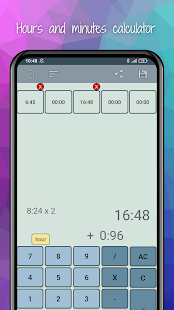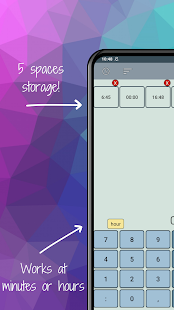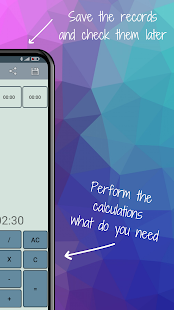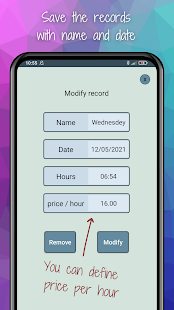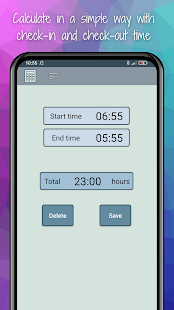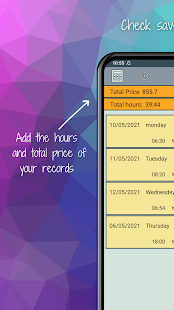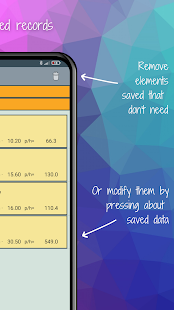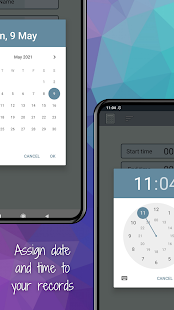This hours calculator: timesheet work hours tracker is a salary and paycheck calculator and time calculator to manage work times.

Use our time calculator + − × ÷ to check the time sheet in work. Useful to calc the paycheck calculator or which work is better salary to choose.

With this hours tracker for work: time-calc you can store the records by date, calculate the total paycheck or salary. In this case, our paycheck calculator is perfect to use the time calculator + − × ÷ and choose your job or calculate the paycheck with our salary calculator.

Examples of the hours calculator: timesheet work hours tracker - salary, paycheck and time calculator:

Time tracker entry: 07:02
Time tracker exit: 16:58
Paycheck hours: 11.57
Salary result with the paycheck calculator: 114.93
Time sheet result with the time tracker: 09:56
This is only a example that you can do with this time calculator or timesheet work hours tracker. Need to know the salary or wage?Use our paycheck calculator and the time calculator × to know if your tsheets are correct.

This time calculator + − × ÷ is designed to easy to control your work for hours tracker or tsheets and know your salary with our paycheck calculator.

Controlling overtime with paycheck calculator in the work will be easier with this hours tracker - time calculator. You will be able to know the salary and the paycheck calculator.
With our time calculator you can count the tsheets or clock in and out with the hours calculator: timesheet work hours tracker.
Use the Timesheet work hours tracker : Time Calc and the paycheck calculator or salary to set an time card of entry and exit, the hours calculator or time calculator - paycheck calculator will calculate it for you.
This time calculator + − × ÷ is perfect for workers to calculate in their work the paycheck. With this time tracker: salary, paycheck and time tracker you can calculate your tsheets, paycheck and salary easily.
This hours tracker is a time calculator will also help us with between times. See some example of calculations with our Hours Calculator: Timesheet work hours tracker - Salary and Time Calculator:
Hours calc for work and salary calculator:
Time tracker: 69 minutes +82 minutes +3:03 hours calculator = 5:34
or
Time Calculator: 100 minutes +5:42 hours tracker +72 minutes = 8:34
This time calculator it works well for part works and know at the end of the day the total tsheets and salary, this is a tsheets and a great hours tracker. We multiply it by the hours and minutes of work or timesheet work hours tracker paycheck and we will obtain the wage of the work day.

Hours Calc: Salary Calculator and Time Calculator, timesheet work hours tracker: Do you know how many hours are 724 minutes? With our time-calculator you will know that it's 12:04. With this Hours Calculator: Timesheet work hours tracker - Salary calculator and Time Calculator it is very easy. Do not waste time with calculations of complex hours tracker or time calculator: tsheets - paycheck calculator - Time Calculator is easy.

It is useful to calc time in companies with many employees. With this hours calculator: Timesheet work hours tracker - Time Calc and paycheck calculator you will have it controlled.

This Hours tracker: Timesheet work hours - Salary calc and time calculator + − × ÷ has many uses:

- Time-calculator: Calculate Cumulative study hours.
- Salary calculator for my workers
- Time tracker: The best tsheets app for hour track.
- Hours Calculator: Calculate time I've lost in traffic jams.
- Paycheck Calculator that accumulate breaks at work.
- Time tracker × : Calculate time of exercise per week.
- Hours calc: Calculate the salary or paycheck of your different jobs.
- Timesheet work hours tracker: Calculate time I do in my work for hours calculator.
- Work time tracker in my different jobs.
- Hours calc and costpoint time and expense: How long does it take to make a project.

Thanks for use our Timesheet work hours tracker: Time Calc and paycheck calc!

### Video

Bug fixes We add functionalities to the calculator hours and minutes.

### Other versions

4.0 released on 10 October 2021 (484 days ago)
3.0 released on 23 May 2021 (624 days ago)
2.10 released on 17 September 2020 (872 days ago)
2.00 released on 23 April 2020 (1019 days ago)

### Details and Recent Ratings

Size: 0 MB
Version: 4.5 by TrasCo Studios
Updated: 05 April 2022 (307 days ago)
Released: 20 October 2019
Installations: more than 100 000
5 Stars: 396
4 Stars: 33
3 Stars: 44
2 Stars: 44
1 Star: 99

### Similar apps

Android Game Categories
Android App Categories